# Explain in quantitative terms the circumstances under which the following reaction can proceed. What would the...

Explain in quantitative terms the

circumstances under which the following

reaction can proceed.

What would the ratio of [product] to [reactant] have to be

for the reaction to proceed spontaneously?

Citrate

isocitrate



G'

° = +13.3 kJ/mol

Citrate --------> isocitrate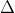G = -RTlnKeq

13300 = -8.314*298*2.303logKeq

logKeq   = -13300/5705.8483

=-2.331

logKeq   = -2.331

Keq    = 10-2.331   = 0.00466

Keq    = [isocitate]/[citrate]

0.00466 = [isocitate]/[citrate]

#### Earn Coin

Coins can be redeemed for fabulous gifts.

Similar Homework Help Questions
• ### 4. The conversion of citrate to isocitrate was found to have a AG" of 6.4 kJ/mol...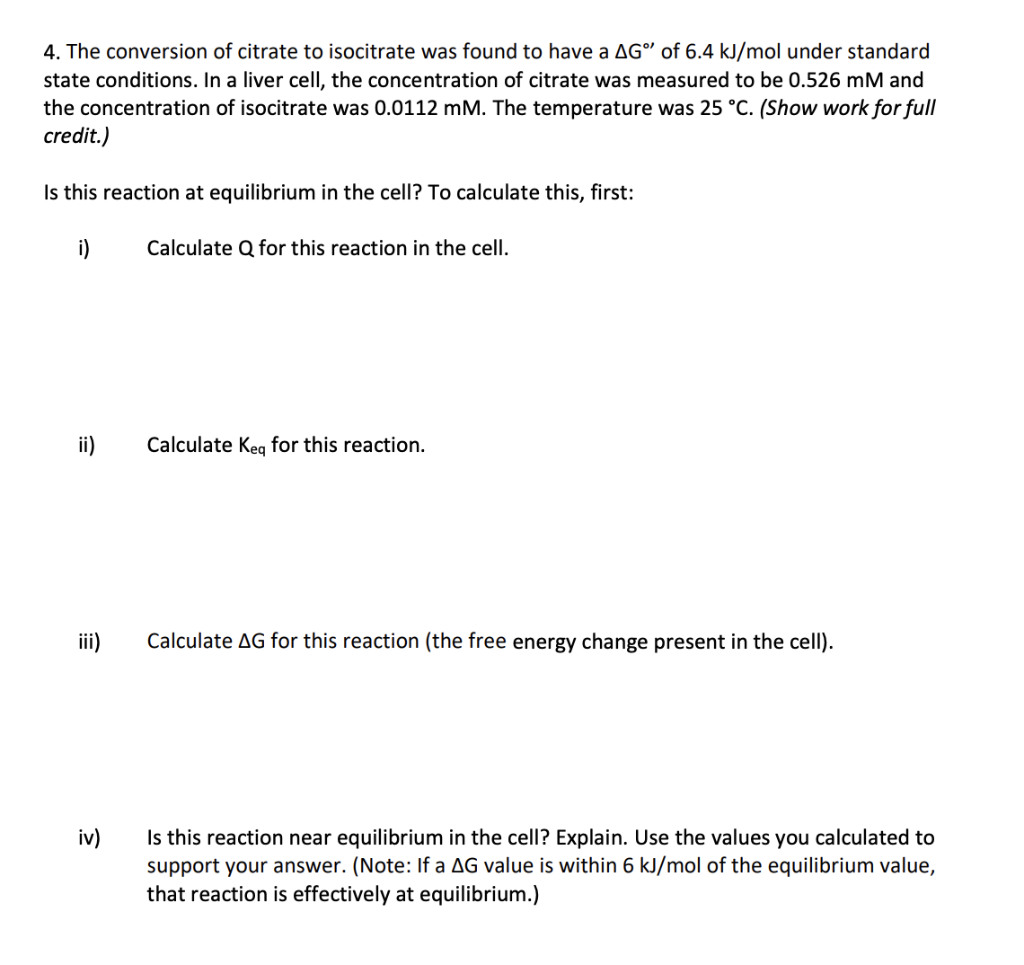4. The conversion of citrate to isocitrate was found to have a AG" of 6.4 kJ/mol under standard state conditions. In a liver cell, the concentration of citrate was measured to be 0.526 mM and the concentration of isocitrate was 0.0112 mM. The temperature was 25 °C. (Show work for full credit.) Is this reaction at equilibrium in the cell? To calculate this, first: i) Calculate Q for this reaction in the cell. ii) Calculate Keq for this reaction. Calculate...

• ### I the AGⓇ of the reaction ABS. 56 kJ/mol which of the following statements are correct?...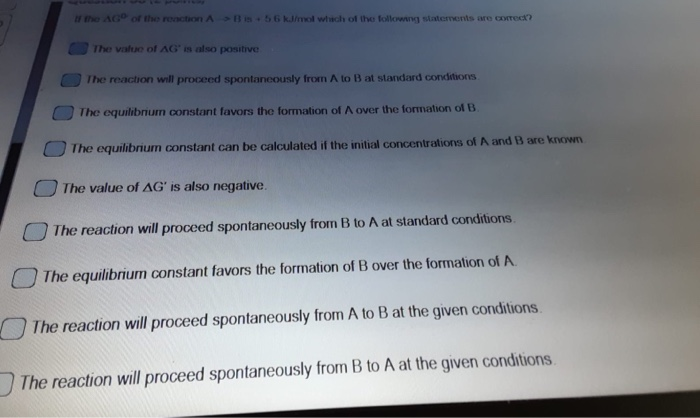I the AGⓇ of the reaction ABS. 56 kJ/mol which of the following statements are correct? The value of AG' is also positive The reaction will proceed spontaneously from A to B at standard conditions The equilibrium constant favors the formation of over the formation of B The equilibrium constant can be calculated if the initial concentrations of A and B are known The value of AG' is also negative. The reaction will proceed spontaneously from B to A at...

• ### help with these please When the following equation is balanced properly under basic conditions, what are...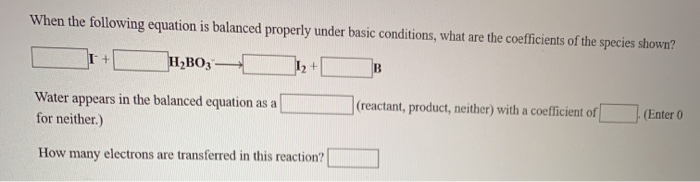help with these please When the following equation is balanced properly under basic conditions, what are the coefficients of the species shown? + H2B03 — 12+ B reactant, product, neither) with a coefficient of (Enter 0 Water appears in the balanced equation as a for neither.) How many electrons are transferred in this reaction? When the following equation is balanced properly under basic conditions, what are the coefficients of the species shown? (reactant, product, neither) with a coefficient of Enter...

• ### organic chemistry answer is D. Can someone please explain why? 3. Which reaction would proceed the...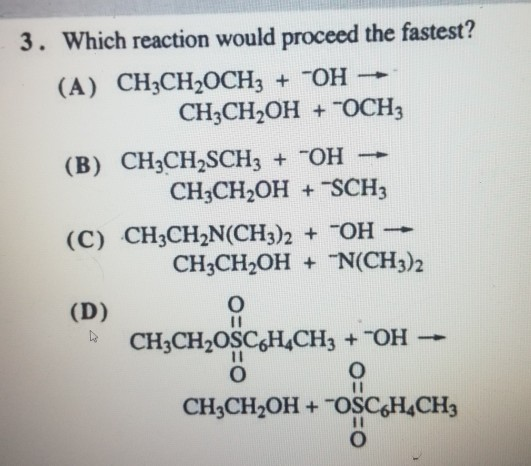organic chemistry answer is D. Can someone please explain why? 3. Which reaction would proceed the fastest? (A) CH3CH2OCH2 + OH → CH3CH2OH + COCH3 (B) CH2CH2SCHz + OH → CH3CH2OH + SCH; (C) CH3CH2N(CH3)2 + OH -- CH3CH2OH + "N(CH3)2 (D) O CH3CH2OSC6H.CH3 + OH - © CHỊCH,OH + OSCH,CH

• ### For the reaction below 2 PC13 (9) + 0,09) = 2POCI; (g) 1) ZVOITZU BAI-T209 AH°: -508.3 kJ/mol AS = -178.3 /mol K o...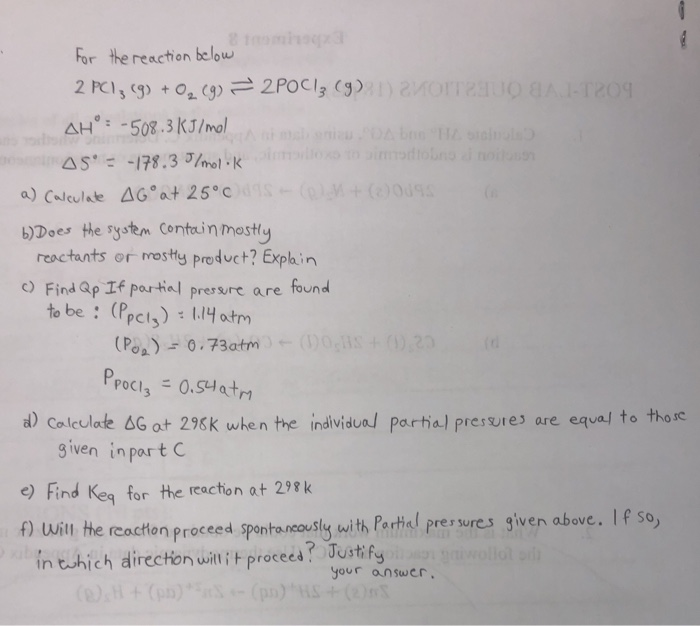For the reaction below 2 PC13 (9) + 0,09) = 2POCI; (g) 1) ZVOITZU BAI-T209 AH°: -508.3 kJ/mol AS = -178.3 /mol K o rongo a) Calculate AG at 25°C + 009 6) Does the system contain mostly reactants or mostly product? Explain () Find Qp If partial pressure are found to be : (Ppcl): 1.14 atm (POR) - 0.73atm - (DOHS + 27 d PPoci = 0.54 atm a) Calculate G at 298k when the individual partial pressures are...

• ### Consider the following reversible reaction: A(g) + 3B(g)  ⇌ AB3(g)   \DeltaΔH = -245 kJ/mol On your Calcs...

Consider the following reversible reaction: A(g) + 3B(g)  ⇌ AB3(g)   \DeltaΔH = -245 kJ/mol On your Calcs sheet, please do the following: a) write the expression for the equilibrium constant for this reaction. b) discuss what effect (if any) would EACH of the following procedures have on the equilibrium, and explain the reason(s.) In here, choose the option that would cause a change in the equilibrium constant. Increasing the volume of the reaction container. Increasing the concentration of product AB3   Increasing...

• ### By which mechanism would the following reaction proceed? please explain answer of why each is wrong or right. Thank you....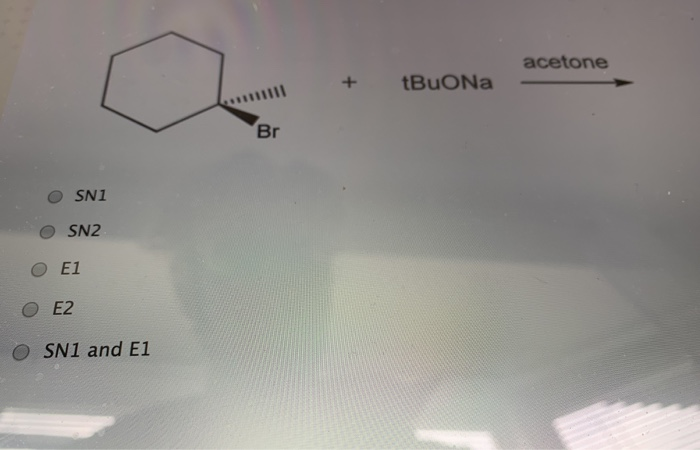By which mechanism would the following reaction proceed? please explain answer of why each is wrong or right. Thank you. acetone . + L + BUONa Buona acetone Br OSNI OSN2 Ο Ε1 O E2 OSN1 and E1

• ### Which of the following reactions would occur the fastest AND under what mechanism would the reaction...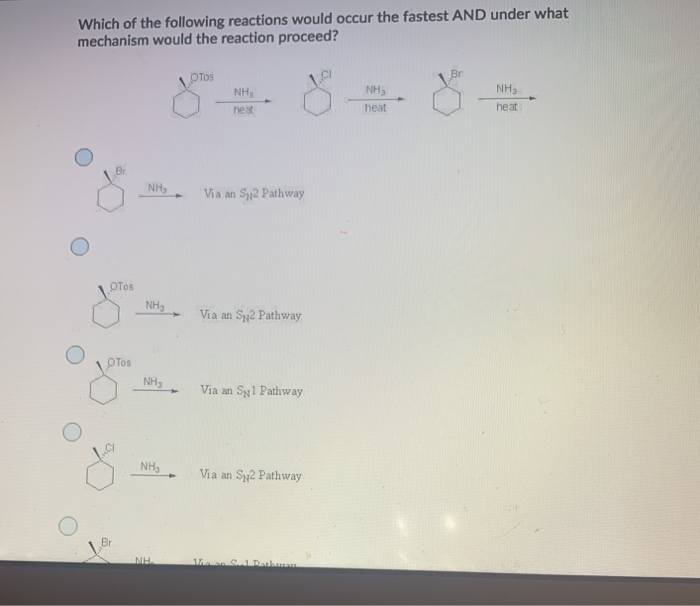Which of the following reactions would occur the fastest AND under what mechanism would the reaction proceed? Tos Br NHS NH, heat NH heat heat B NH Via an Sy2 Pathway OTos NHS Via an Sy2 Pathway Tos NHS Via an Spi Pathway O NH Via an Sy2 Pathway Br M.

• ### For the following reaction, please answer the following questions: A) Which type of substitution reaction would...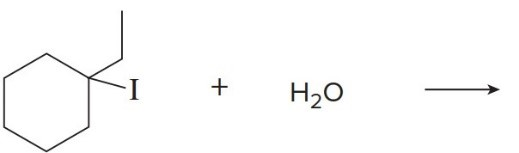For the following reaction, please answer the following questions: A) Which type of substitution reaction would it take place, SN1 or SN2? B) Does it have a good leaving group? How do you know? C) What is the intermediate? D) Show electron move using arrows. E) Show product structure. Indicate stereochemistry of the product comparing to the reactant. OH + I-

• ### 1. Define the following terms: a. Activation Energy b. Exothermic Reaction 2. Explain what Le Chatelier's...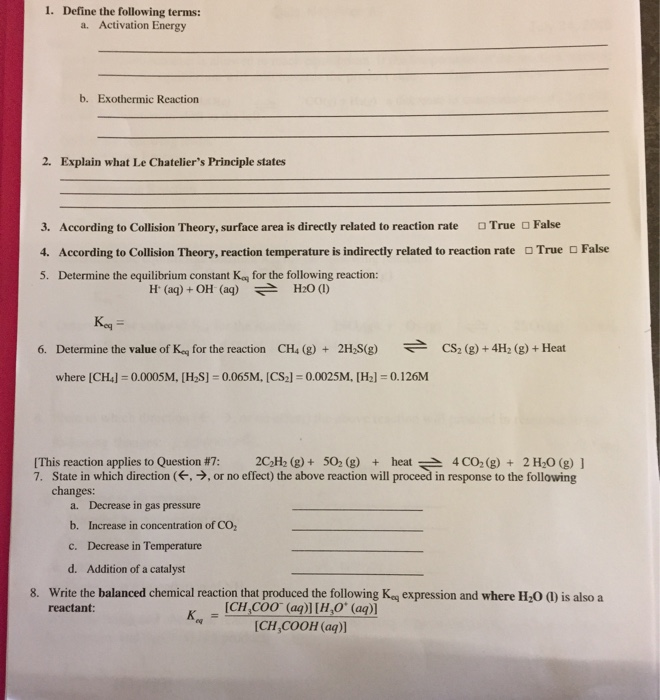1. Define the following terms: a. Activation Energy b. Exothermic Reaction 2. Explain what Le Chatelier's Principle states False True False 3. According to Collision Theory, surface area is directly related to reaction rate True 4. According to Collision Theory, reaction temperature is indirectly related to reaction rate 5. Determine the equilibrium constant K for the following reaction: H+ (aq) + OH(aq) = H20 (1) Keg 6. Determine the value of Key for the reaction CH4 (g) + 2H S(g)...